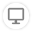405
Views
7
Solved
###### [Excel Package] Calculate Formulae
QuestionForge component by Carlos Alfaro

Hi,

Is this extension able to calculate cells with formulae?
EPPlus has a function to do this: Calculate.

Cheers,
Eduardo JauchCarlos Alfaro
MVP
Solution

Hi again Eduardo,

I've uploaded a new version of the component, with three new actions:

• Workbook_Calculate() - calculates all formulas in the Workbook
• Worksheet_Calculate() - calculates all formulas in one Worksheet
• Cell_CalculateByIndex() - calculates the formula of a cell, defined by its index

Thank you for your request and hope this helps!

CACarlos Alfaro
MVP

Hi Eduardo,

I will evaluate the possibility of adding the Calculate functionality to the component.

Thank you for the request!

CACarlos Alfaro
MVP
Solution

Hi again Eduardo,

I've uploaded a new version of the component, with three new actions:

• Workbook_Calculate() - calculates all formulas in the Workbook
• Worksheet_Calculate() - calculates all formulas in one Worksheet
• Cell_CalculateByIndex() - calculates the formula of a cell, defined by its index

Thank you for your request and hope this helps!

CAEduardo Jauch
MVP

Thanks! It will be useful! :)Vibhu Gupta

Hi,

Please pardon the noob question... how do i add a formula to a cell?

When i write a formula, it just displays as text in the excel, the calculate function doesn't seem to do anything.

ThanksCarlos Alfaro
MVP

Hello Vibhu,

Thank you for this request, I've uploaded a new version of the component, with the ability to write a formula to a Cell identified by index or name.

Thank you.

CAMaple Field

Hello Carlos, do you have any example of setting formula in the excel cell? I tried but it it will display "#value!" calculation error after the excel file is generated. Then, when I open the excel file and click "Enable Editing", the formulas work well.

Any tricks?

Maple FieldSai Charan

Hi Carlos,

Is it possible to read the excel with headers starting from row 12 instead of row 1?

Thanks,

Saicharan

Community GuidelinesBe kind and respectful, give credit to the original source of content, and search for duplicates before posting.